Loops checkup #3
What will this code print (assume / means a new line) *
1 point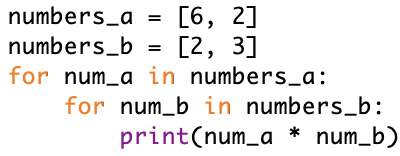What will this code print (assume / means a new line) *
1 point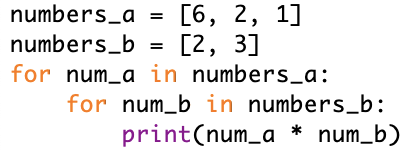What will this code print (assume / means a new line) *
1 point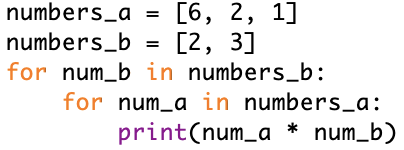What will this code print (assume / means a new line) *
1 point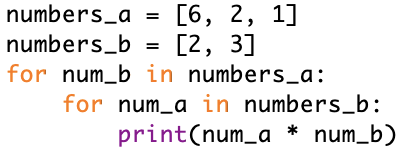What will this code print (assume / means a new line). Remember that % is the rest operation, for example 6 % 3 = 0; 3 % 6 = 3; 3 % 2 = 1. Also remember that for an if statement, 0 is False and any other number is True. *
1 point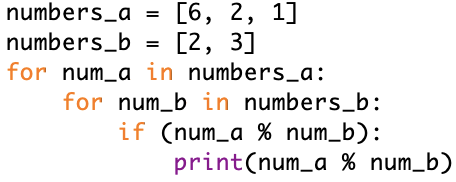What will this code print (assume / means a new line) *
1 point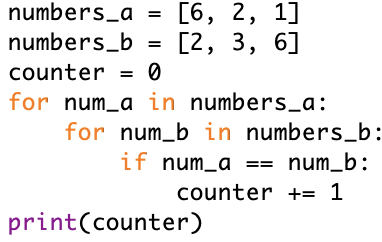This content is neither created nor endorsed by Google. Report Abuse - Terms of Service